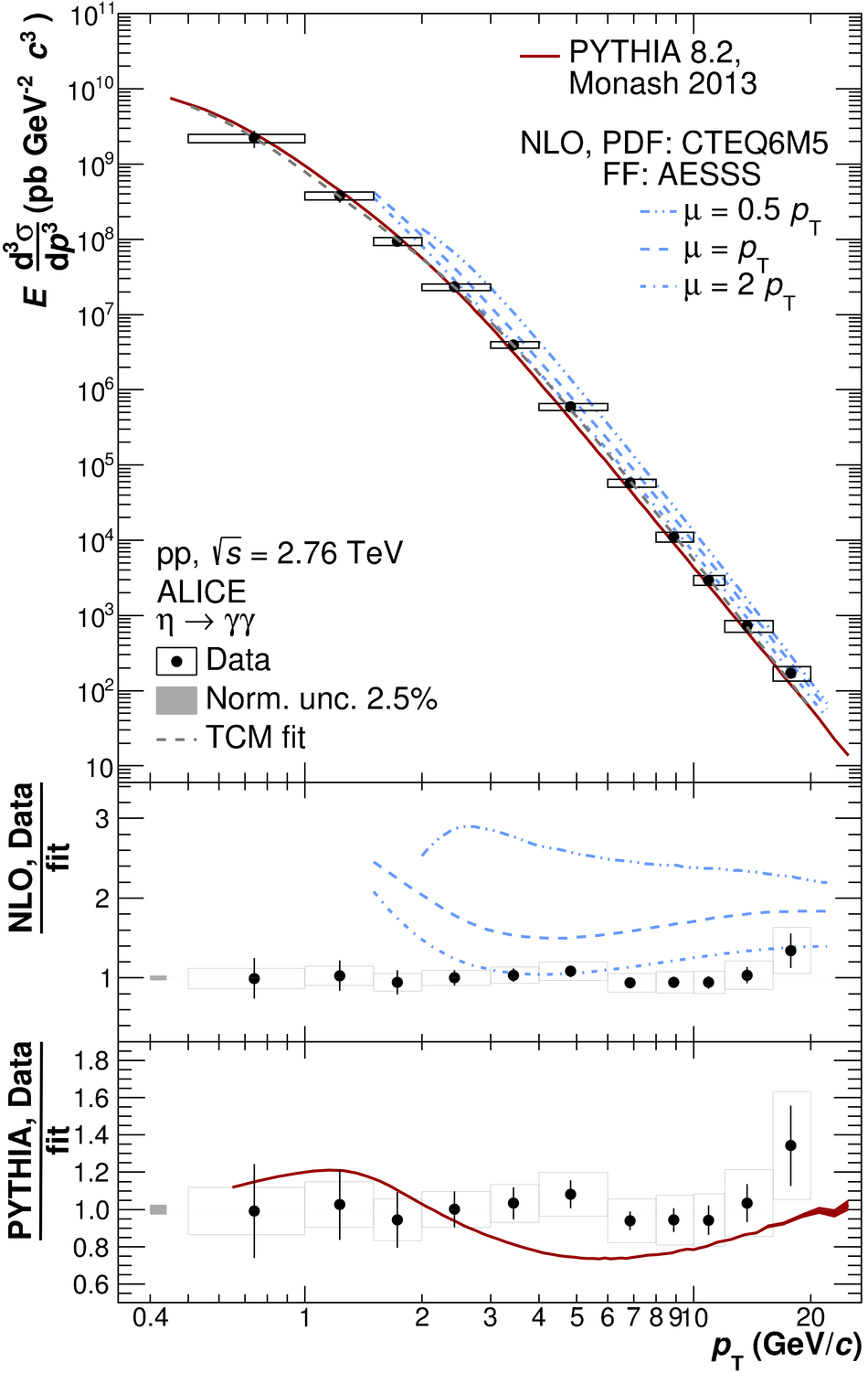# Figure 9

 Invariant differential cross section of the $\pi^0$ (left, top panel) and $\eta$ meson (right, top panel) for pp collisions at $\sqrt{s} = 2.76$ TeV The data are compared to PYTHIA 8.2 generator-level simulations using the Monash 2013 tune as well as recent NLO pQCD calculations [3,6]. The ratios of the data and the calculations to the respective two-component model fits [25,26] to the data are shown in the lower panels The horizontal error bars denote statistical, the boxes systematic uncertainties.